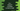# C++ program to check if a number is even or odd## C++ program to check if a number is even or odd:

In this post, we will learn how to check if a user-given number is even or odd.

This program will ask the user to enter a number. It will check if the number is even or odd and it will print one message.

With this program, you will learn how to read user inputs, how to do basic mathematical calculations, and how to print messages to the user.

### What are even and odd numbers:

A number is called an even number if it can be divided into two equal whole numbers. Or, we can say that if a number can be exactly divided by 2, it is an even number. Otherwise, it is an odd number.

### How to find if a number can be exactly divisible by 2:

We can use the modulo operator to check if a number is exactly divisible by 2 or not. The modulo operator, % can be defined as a % b, and it returns the remainder of a/b. So, if a number is exactly divisible by 2, the value of number % 2 will be 0.

So, the program will read a number as input from the user, check if it is exactly divisible by 2 or not by using the modulo operator, and print one message back.

## Method 1: C++ program to check if a user-given number is odd or even:

Below is the complete C++ program:

``````#include <iostream>
using namespace std;

int main()
{
int num;

cout << "Enter a number : " << endl;
cin >> num;

if (num % 2 == 0)
{
cout << "The entered number is Even" << endl;
}
else
{
cout << "The entered number is Odd" << endl;
}
}``````

Here,

• num is an integer variable.
• It is asking the user to enter a number. We are using cout to print that message to the user.
• Using cin, we are reading the user input number and storing it in num.
• The if-else block is checking if num is even or odd. Based on that, we are printing one message.

### Output:

If you run this program, it will print output as like below:

``````Enter a number :
10
The entered number is Even

Enter a number :
13
The entered number is Odd``````

As you can see here, it takes one number from the user, and prints if the number is odd or even.

## Method 2: C++ program to check if a user-given number is odd or even by using ternary operator:

We can also use the ternary operator to check if a user-given number is odd or even and print that message to the user.

Let me show you how it works:

``````#include <iostream>
using namespace std;

int main()
{
int num;

cout << "Enter a number : " << endl;
cin >> num;

string result = (num % 2 == 0) ? "The entered number is Even" : "The entered number is Odd";

cout << result << endl;
}``````

The ternary operator works similarly to the if-else statement. It can be defined as like below:

``condition ? expression_1 : expression_2``

If the condition returns true, it will execute the expression_1, else it will execute expression_2.

In the above example, we are checking the value of num % 2 is 0 or not. Based on its value, we are assigning a string to result. The last line is printing the value of result.

If you run this program, it will print similar output:

``````Enter a number :
12
The entered number is Even

Enter a number :
11
The entered number is Odd``````